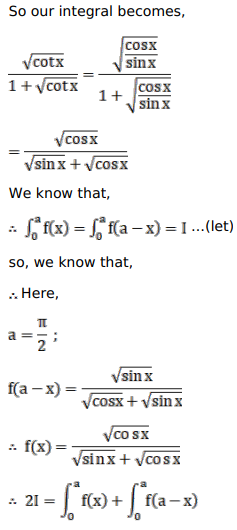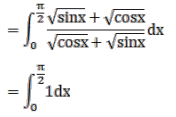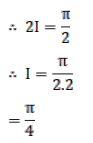# Mark against the correct answer in the following:

Question:

Mark $(\sqrt{)}$ against the correct answer in the following:

$\int_{0}^{\pi / 2} \frac{\sqrt{\cot x}}{(1+\sqrt{\cot x})} d x=?$

A. $\frac{\pi}{4}$

B. $\frac{\pi}{2}$

C. 0

D. 1

Solution: